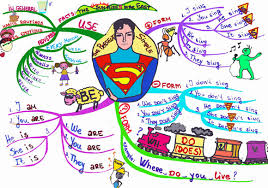Present Simple, questions
ПІБ:
Клас:
Дата:
1.а)

do

б)

does

в)

is

г)

am

д)

are

2.

Where__ his brother live?

а)

do

б)

does

в)

is

г)

am

д)

are

3.

Who__ in the park?

а)

do

б)

does

в)

am

г)

is

д)

are

4.

What class __ we in?

а)

do

б)

does

в)

am

г)

is

д)

are

5.

а)

do

б)

does

в)

am

г)

is

д)

are

6.

Where __ Ann from?

а)

do

б)

does

в)

am

г)

is

д)

are

7.

What game __ Bob play?

а)

do

б)

does

в)

am

г)

is

д)

are

8.

а)

do

б)

does

в)

am

г)

is

д)

are

9.

What language __ her sister learn?

а)

do

б)

does

в)

am

г)

is

д)

are

10.

Sorry, I___ late.

а)

do

б)

does

в)

am

г)

is

д)

are

11.

We__ good at dancing.

а)

do

б)

does

в)

am

г)

is

д)

are

1. д (1 балів)
2. б (1 балів)
3. г (1 балів)
4. д (1 балів)
5. а (1 балів)
6. г (1 балів)
7. б (1 балів)
8. д (1 балів)
9. б (1 балів)
10. в (1 балів)
11. д (1 балів)